Updating search results...

# 19 Results

View
Selected filters:
• cube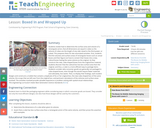Educational Use
Rating
0.0 stars

Students find the volume and surface area of a rectangular box (e.g., a cereal box), and then figure out how to convert that box into a new, cubical box having the same volume as the original. As they construct the new, cube-shaped box from the original box material, students discover that the cubical box has less surface area than the original, and thus, a cube is a more efficient way to package things. Students then consider why consumer goods generally aren't packaged in cube-shaped boxes, even though they would require less material to produce and ultimately, less waste to discard. To display their findings, each student designs and constructs a mobile that contains a duplicate of his or her original box, the new cube-shaped box of the same volume, the scraps that are left over from the original box, and pertinent calculations of the volumes and surface areas involved. The activities involved provide valuable experience in problem solving with spatial-visual relationships.

Subject:
Applied Science
Engineering
Material Type:
Activity/Lab
Lesson Plan
Provider:
TeachEngineering
Provider Set:
TeachEngineering
Author:
Mary R. Hebrank
09/18/2014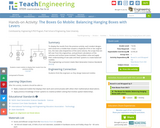Educational Use
Rating
0.0 stars

To display the results from the previous activity, each student designs and constructs a mobile that contains a duplicate of his or her original box, the new cube-shaped box of the same volume, the scraps that are left over from the original box, and pertinent calculations of the volumes and surface areas involved. They problem solve and apply their understanding of see-saws and lever systems to create balanced mobiles.

Subject:
Applied Science
Engineering
Material Type:
Activity/Lab
Provider:
TeachEngineering
Provider Set:
TeachEngineering
Author:
Mary R. Hebrank
10/14/2015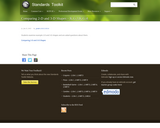Rating
0.0 stars

In this assessment students are asked to compare 2-D and 3-D shapes. Students will be given a rectangle and a cube. Students are asked whether the shapes are 2-D or 3-D and whether they are flat or solid. Students will use a Venn Diagram to compare and contrast the attributes of the shapes. An assessment sheet and rubric are provided.

Subject:
Mathematics
Material Type:
Activity/Lab
Provider:
Hawaii State Department of Education
Author:
Hawaii State Department of Education
02/26/2019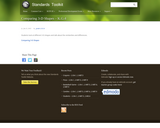Rating
0.0 stars

In this assessment task students are asked to use a Venn Diagram to compare 3-D solid shapes. Students are given a cube and a cylinder. They are asked how the shapes are the same and how they are different. Teachers can dictate student responses. An assessment sheet and rubric are provided.

Subject:
Mathematics
Material Type:
Activity/Lab
Provider:
Hawaii State Department of Education
Author:
Hawaii State Department of Education
02/26/2019Rating
0.0 stars

This three-part example from Illuminations highlights different aspects of students' understanding and use of patterns as they analyze relationships and make predictions, as discussed in the Algebra Standard. This first part, Making Patterns, includes an interactive figure for creating, comparing, and viewing multiple repetitions of patterns. The interactive figure illustrates how students can create pattern units of squares, then predict how patterns with different numbers of squares will appear when repeated in a grid, and check their predictions. In the second part, Describing Patterns, examples of various ways students might interpret the same sequence of cubes are given. This illustrates the importance of discussing and analyzing patterns in the classroom. The third part, Extending Pattern Understandings, demonstrates ways in which students begin to create a 'unit of units,' or a grouping that can be repeated, and begin to relate two patterns in a functional relationship. e-Math Investigations are selected e-examples from the electronic version of the Principles and Standards of School Mathematics (PSSM). The e-examples are part of the electronic version of the PSSM document. Given their interactive nature and focused discussion tied to the PSSM document, the e-examples are natural companions to the i-Math investigations.

Subject:
Mathematics
Material Type:
Activity/Lab
Interactive
Lesson Plan
Provider:
National Council of Teachers of Mathematics
Author:
National Council of Teachers of Mathematics
02/26/2019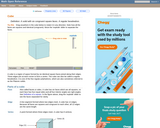Rating
0.0 stars

An interactive applet and associated web page that demonstrate the properties of a cube. A 3-D cube is shown in the applet which can be interactively manipulated using the mouse. Research has shown that some younger students have difficulty visualizing the parts of a 3D object that are hidden. To help with this, the student can rotate the cube in any axis simply by dragging it with the mouse. It can also be 'exploded' - where a slider gradually separates the faces to reveal the ones behind. The cube can also be made translucent so you see through it to the other side. Applet can be enlarged to full screen size for use with a classroom projector, and printed to make handouts. This resource is a component of the Math Open Reference Interactive Geometry textbook project at http://www.mathopenref.com.

Subject:
Geometry
Mathematics
Material Type:
Simulation
Provider:
Math Open Reference
Author:
John Page
05/07/2019Rating
0.0 stars

Students explore the volume of a box based on the amount of unit cubes that can fit inside of it in this student interactive, from Illuminations. They are prompted to come up with a rule for determining the volume of a box when its width, depth, and height are known.

Subject:
Geometry
Mathematics
Material Type:
Activity/Lab
Interactive
Simulation
Provider:
National Council of Teachers of Mathematics
Author:
Illuminations
02/26/2019Rating
0.0 stars

Students to explore various geometric solids and their properties in this student interactive, from Illuminations. Students can virtually manipulate and color each solid to investigate properties such as the number of faces, edges, and vertices. Students can explore tetrahedrons, cubes, octahedrons, dodecahedrons, icosahedrons, and irregular polyhedrons by toggling between solid and net to see different views of the selected shape.

Subject:
Geometry
Mathematics
Material Type:
Activity/Lab
Interactive
Provider:
National Council of Teachers of Mathematics
Author:
Illuminations
02/26/2019Conditional Remix & Share Permitted
CC BY-NC
Rating
0.0 stars
Rating
0.0 stars
Subject:
Mathematics
Material Type:
Full Course
Provider:
Pearson
11/02/2020Conditional Remix & Share Permitted
CC BY-NC
Rating
0.0 stars

Surface Area and Volume

Type of Unit: Conceptual

Prior Knowledge

Students should be able to:

Identify rectangles, parallelograms, trapezoids, and triangles and their bases and heights.
Identify cubes, rectangular prisms, and pyramids and their faces, edges, and vertices.
Understand that area of a 2-D figure is a measure of the figure's surface and that it is measured in square units.
Understand volume of a 3-D figure is a measure of the space the figure occupies and is measured in cubic units.

Lesson Flow

The unit begins with an exploratory lesson about the volumes of containers. Then in Lessons 2–5, students investigate areas of 2-D figures. To find the area of a parallelogram, students consider how it can be rearranged to form a rectangle. To find the area of a trapezoid, students think about how two copies of the trapezoid can be put together to form a parallelogram. To find the area of a triangle, students consider how two copies of the triangle can be put together to form a parallelogram. By sketching and analyzing several parallelograms, trapezoids, and triangles, students develop area formulas for these figures. Students then find areas of composite figures by decomposing them into familiar figures. In the last lesson on area, students estimate the area of an irregular figure by overlaying it with a grid. In Lesson 6, the focus shifts to 3-D figures. Students build rectangular prisms from unit cubes and develop a formula for finding the volume of any rectangular prism. In Lesson 7, students analyze and create nets for prisms. In Lesson 8, students compare a cube to a square pyramid with the same base and height as the cube. They consider the number of faces, edges, and vertices, as well as the surface area and volume. In Lesson 9, students use their knowledge of volume, area, and linear measurements to solve a packing problem.

Subject:
Geometry
Mathematics
Provider:
Pearson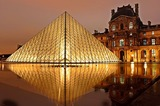Conditional Remix & Share Permitted
CC BY-NC
Rating
0.0 stars

Lesson OverviewStudents use scissors to transform a net for a unit cube into a net for a square pyramid. They then investigate how changing a figure from a cube to a square pyramid affects the number of faces, edges, and vertices and how it changes the surface area and volume.Key ConceptsA square pyramid is a 3-D figure with a square base and four triangular faces.In this lesson, the net for a cube is transformed into a net for a square pyramid. This requires cutting off one square completely and changing four others into isosceles triangles.It is easy to see that the surface area of the pyramid is less than the surface area of the cube, because part of the cube's surface is cut off to create the pyramid. Specifically, the surface area of the pyramid is 3 square units, and the surface area of the cube is 6 square units. Students will be able to see visually that the volume of the pyramid is less than that of the cube.Students consider the number of faces, vertices, and edges of the two figures. A face is a flat side of a figure. An edge is a segment where 2 faces meet. A vertex is the point where three or more faces meet. A cube has 6 faces, 8 vertices, and 12 edges. A square pyramid has 5 faces, 5 vertices, and 8 edges.Goals and Learning ObjectivesChange the net of a cube into the net of a pyramid.Find the surface area of the pyramid.

Subject:
Geometry
Material Type:
Lesson Plan
Author:
Pearson
11/02/2020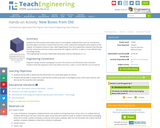Educational Use
Rating
0.0 stars

Students find the volume and surface area of a rectangular box (e.g., a cereal box), and then figure out how to convert that box into a new, cubical box having the same volume as the original. As they construct the new, cube-shaped box from the original box material, students discover that the cubical box has less surface area than the original, and thus, a cube is a more efficient way to package things.

Subject:
Applied Science
Engineering
Material Type:
Activity/Lab
Lesson Plan
Provider:
TeachEngineering
Provider Set:
TeachEngineering
Author:
Mary R. Hebrank
01/20/2009Rating
0.0 stars

In this assessment task students will become construction engineers and build three-dimensional shapes out of straws, twist ties, paper clips, toothpicks and other supplies. An assessment task sheet and rubric are provided.

Subject:
Mathematics
Material Type:
Activity/Lab
Provider:
Hawaii State Department of Education
Author:
Hawaii State Department of Education
02/26/2019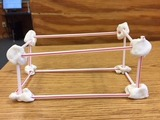Unrestricted Use
CC BY
Rating
0.0 stars

This resource is from Tools4NCTeachers.&nbsp;&nbsp;In this lesson, students explore 3D shapes, such as rectangular prisms and cubes. The students will identify the attributes for each 3D shape and build shapes using sticks, marshmallows, and other materials.&nbsp;&nbsp;Pictures of sample student work and printable recording sheets are provided within this lesson.

Subject:
Mathematics
Material Type:
Activity/Lab
Lesson
Lesson Plan
Author:
DAWNE COKER
06/24/2020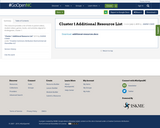Conditional Remix & Share Permitted
CC BY-NC-SA
Rating
0.0 stars

This resource is part of Tools4NCTeachers.

This list contains links to parent videos, student videos, games, books, and activities aligned to Kindergarten, Cluster 1.

Subject:
Mathematics
Material Type:
Activity/Lab
Game
11/06/2019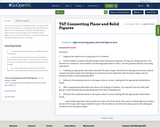Conditional Remix & Share Permitted
CC BY-NC-SA
Rating
0.0 stars

This resource is part of Tools4NCTeachers.

The goal of this lesson is for students to understand that plane figures are different from solid figures. Students will identify and locate the face of a solid figure that matches a plane figure, such as the face of a cube is a square, the base of a triangular based pyramid is a triangle.

Subject:
Mathematics
Material Type:
Lesson
Lesson Plan
11/06/2019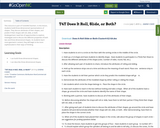Conditional Remix & Share Permitted
CC BY-NC-SA
Rating
0.0 stars

This resource is part of Tools4NCTeachers.

In this lesson, students build three-dimensional representations of shapes. They discuss attributes of their shapes, and predict if their shapes will roll, slide, or both.

Kindergartners need lots of opportunities to explore, investigate and to discuss the different properties of both two and three dimensional shapes. As students learn more geometric content, they will begin to be more sophisticated in their ways of thinking.

Subject:
Mathematics
Material Type:
Lesson
Lesson Plan
11/06/2019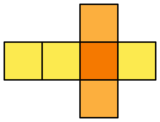Unrestricted Use
CC BY
Rating
0.0 stars

This resource is from Tools4NCTeachers.In this lesson, students are given boxes to cut apart.&nbsp; As they decompose the boxes, students notice the 2-dimensional shapes used to construct their 3-dimensional boxes.&nbsp; A printable student recording sheet is provided within this lesson.Remix to include pictures of student work samples.&nbsp;

Subject:
Mathematics
Material Type:
Activity/Lab
Lesson
Lesson Plan
Author:
DAWNE COKER
06/24/2020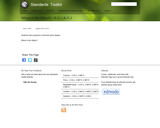Rating
0.0 stars

In this assessment task students will determine the position of a shape from the perspective of another figure. They will be asked to use their words to tell where an object is and to tell the shape of the object they found. An assessment task sheet and a rubric are provided.

Subject:
Mathematics
Material Type:
Activity/Lab
Provider:
Hawaii State Department of Education
Author:
Hawaii State Department of Education